LEGACY CONTENT. If you are looking for Voteview.com, PLEASE CLICK HERE

This site is an archived version of Voteview.com archived from University of Georgia on May 23, 2017. This point-in-time capture includes all files publicly linked on Voteview.com at that time. We provide access to this content as a service to ensure that past users of Voteview.com have access to historical files. This content will remain online until at least January 1st, 2018. UCLA provides no warranty or guarantee of access to these files.

### 45-733 PROBABILITY AND STATISTICS I

A SHORT GUIDE TO HYPOTHESIS TESTING

1. Ho: m = mo
H1: m = m1 > mo

Test: If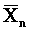> mo + c then reject Ho

1. To find c when s2 is known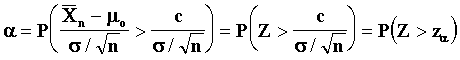To solve for c (a) fix a (c) and use Z table.

2. To find c when s2 is not known, large sample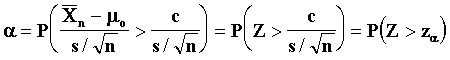To solve for c (a) fix a (c) and use Z table.

3. To find c when s2 is not known, small sample from N(m, s2)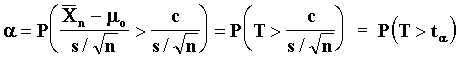Where T has a t distribution with n - 1 degrees of freedom. To solve for c (a) fix a (c) and use t table.

2. Ho: m = mo
H1: m ¹ mo

Test: If> mo + c or -< mo - c then reject Ho.

1. To find c when s2 is known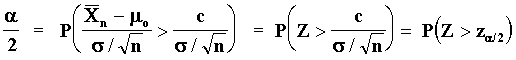To solve for c (a) fix a (c) and use Z table.

2. To find c when s 2 is not known, large sample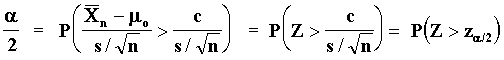To solve for c (a) fix a (c) and use Z table.

3. To find c when s2 is not known, small sample from N(m , s2)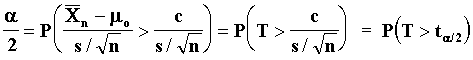Where T has a t distribution with n - 1 degrees of freedom. To solve for c (a) fix a (c) and use t table.

3. Ho: m 1 - m2 = 0
H1: m1 - m2 ¹ 0

Test: If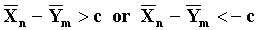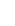then reject Ho.

1. To find c when s12 and s22 are known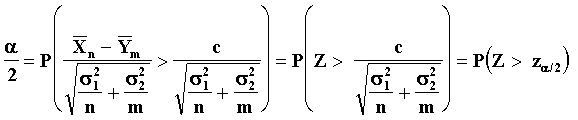To solve for c (a) fix a (c) and use Z table.

2. To find c when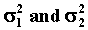are not known, large sample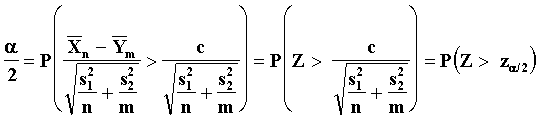To solve for c (a) fix a (c) and use Z table.

3. To find c whenare not known, small samples from N(m , s2)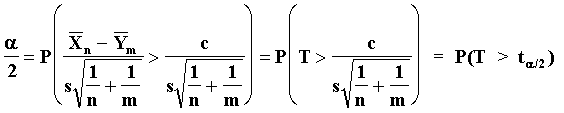Where T has a t distribution with n + m - 2 degrees of freedom and s2 is given by: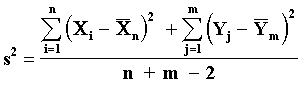To solve for c (a) fix a (c) and use t table.

4. Ho: s2 =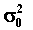H1: s2 =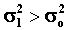Test: If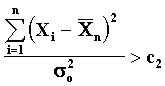then reject Ho.

To find c2 set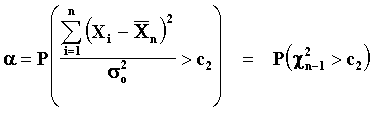and use the chi-square table with n - 1 degrees of freedom.

5. Ho: s2 =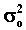H1: s2 =Test: Ifthen reject Ho.

To find c1 and c2 set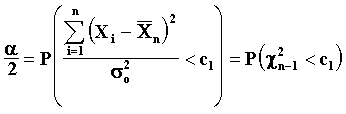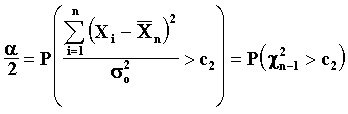and use the chi-square table with n - 1 degrees of freedom.

6. Ho: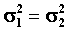H1: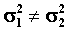Test: If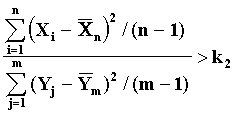or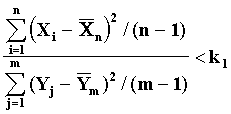then reject Ho. To find k1 and k2 set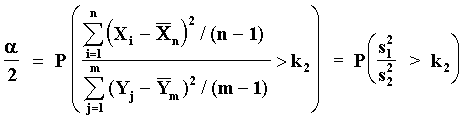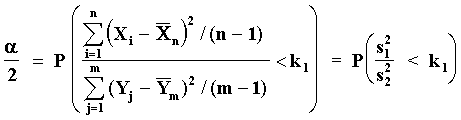Use the F table with n - 1 and m - 1 degrees of freedom to find k2 and then use the reciprocal of the entry for m - 1 and n - 1 degrees of freedom for k1.